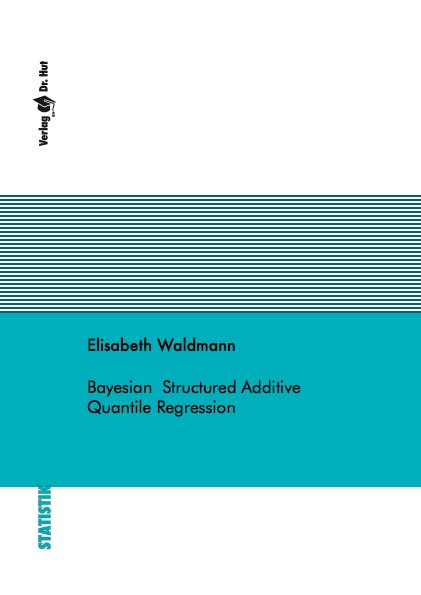Datenbestand vom 20. August 2019aktualisiert am 20. August 2019

# ISBN 9783843912587Euro 72,00 inkl. 7% MwSt

978-3-8439-1258-7, Reihe Statistik

Elisabeth Waldmann
Bayesian Structured Additive Quantile Regression

137 Seiten, Dissertation Georg-August-Universität Göttingen (2013), Softcover, A5

## Zusammenfassung / Abstract

Quantile regression provides a convenient framework for analyzing the impact of covariates on the complete conditional distribution of a response variable instead of only the mean. While frequentist treatments of quantile regression are typically completely nonparametric, a Bayesian formulation relies on assuming the asymmetric Laplace distribution as auxiliary error distribution that yields posterior modes equivalent to frequentist estimates. A location scale mixture of normals representation of this distribution makes it feasible for Markov Chain Monte Carlo (MCMC) Methods.

On this basis four different approaches are developed. The first one is the transfer of different flexible modelling concepts from Gaussian mean regression to Bayesian semiparametric quantile regression. In particular, highdimensional geoadditive models comprising LASSO regularization priors and mixed models with potentially non-normal random effects distribution modeled via a Dirichlet process mixture. The second approach developed in this work is the extension of the above mentioned location scale mixture to a bivariate case. Due to the drawbacks in terms of computational speed in MCMC, the method for the univariate case is then transfered to the variational approximation setting. This approach is based on a slightly different location scale mixture representation. The fourth part is the development of an MCMC algorithm for Bayesian expectile regression, where - instead of the asymmetric Laplace distribution - an asymmetric Gaussian distribution is used as an error distribution. For these four methods the theoretical approach is described, and the implementation is checked by simulation studies and illustrated by data examples from different areas of application.